Electrochemistry

# 100 Review of Redox Chemistry

### Learning Objectives

By the end of this section, you will be able to:

• Describe defining traits of redox chemistry
• Identify the oxidant and reductant of a redox reaction
• Balance chemical equations for redox reactions using the half-reaction method

Since reactions involving electron transfer are essential to the topic of electrochemistry, a brief review of redox chemistry is provided here that summarizes and extends the content of an earlier text chapter (see chapter on reaction stoichiometry). Readers wishing additional review are referred to the text chapter on reaction stoichiometry.

### Oxidation Numbers

By definition, a redox reaction is one that entails changes in oxidation number (or oxidation state) for one or more of the elements involved. The oxidation number of an element in a compound is essentially an assessment of how the electronic environment of its atoms is different in comparison to atoms of the pure element. By this description, the oxidation number of an atom in an element is equal to zero. For an atom in a compound, the oxidation number is equal to the charge the atom would have in the compound if the compound were ionic. Consequential to these rules, the sum of oxidation numbers for all atoms in a molecule is equal to the charge on the molecule. To illustrate this formalism, examples from the two compound classes, ionic and covalent, will be considered.

Simple ionic compounds present the simplest examples to illustrate this formalism, since by definition the elements’ oxidation numbers are numerically equivalent to ionic charges. Sodium chloride, NaCl, is comprised of Na+ cations and Cl anions, and so oxidation numbers for sodium and chlorine are, +1 and −1, respectively. Calcium fluoride, CaF2, is comprised of Ca2+ cations and F anions, and so oxidation numbers for calcium and fluorine are, +2 and −1, respectively.

Covalent compounds require a more challenging use of the formalism. Water is a covalent compound whose molecules consist of two H atoms bonded separately to a central O atom via polar covalent O−H bonds. The shared electrons comprising an O−H bond are more strongly attracted to the more electronegative O atom, and so it acquires a partial negative charge in the water molecule (relative to an O atom in elemental oxygen). Consequently, H atoms in a water molecule exhibit partial positive charges compared to H atoms in elemental hydrogen. The sum of the partial negative and partial positive charges for each water molecule is zero, and the water molecule is neutral.

Imagine that the polarization of shared electrons within the O−H bonds of water were 100% complete—the result would be transfer of electrons from H to O, and water would be an ionic compound comprised of O2− anions and H+ cations. And so, the oxidations numbers for oxygen and hydrogen in water are −2 and +1, respectively. Applying this same logic to carbon tetrachloride, CCl4, yields oxidation numbers of +4 for carbon and −1 for chlorine. In the nitrate ion,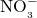, the oxidation number for nitrogen is +5 and that for oxygen is −2, summing to equal the 1− charge on the molecule: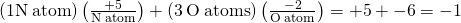### Balancing Redox Equations

The unbalanced equation below describes the decomposition of molten sodium chloride: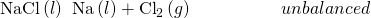This reaction satisfies the criterion for redox classification, since the oxidation number for Na is decreased from +1 to 0 (it undergoes reduction) and that for Cl is increased from −1 to 0 (it undergoes oxidation). The equation in this case is easily balanced by inspection, requiring stoichiometric coefficients of 2 for the NaCl and Na: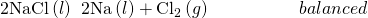Redox reactions that take place in aqueous solutions are commonly encountered in electrochemistry, and many involve water or its characteristic ions, H+(aq) and OH(aq), as reactants or products. In these cases, equations representing the redox reaction can be very challenging to balance by inspection, and the use of a systematic approach called the half-reaction method is helpful. This approach involves the following steps:

1. Write skeletal equations for the oxidation and reduction half-reactions.
2. Balance each half-reaction for all elements except H and O.
3. Balance each half-reaction for O by adding H2O.
4. Balance each half-reaction for H by adding H+.
5. Balance each half-reaction for charge by adding electrons.
6. If necessary, multiply one or both half-reactions so that the number of electrons consumed in one is equal to the number produced in the other.
7. Add the two half-reactions and simplify.
8. If the reaction takes place in a basic medium, add OH ions the equation obtained in step 7 to neutralize the H+ ions (add in equal numbers to both sides of the equation) and simplify.

The examples below demonstrate the application of this method to balancing equations for aqueous redox reactions.

Balancing Equations for Redox Reactions in Acidic Solutions Write the balanced equation representing reaction between solid copper and nitric acid to yield aqueous copper(II) ions and nitrogen monoxide gas.

Solution Following the steps of the half-reaction method:

1. Write skeletal equations for the oxidation and reduction half-reactions.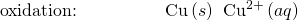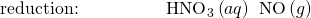2. Balance each half-reaction for all elements except H and O.3. Balance each half-reaction for O by adding H2O.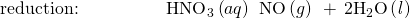4. Balance each half-reaction for H by adding H+.5. Balance each half-reaction for charge by adding electrons.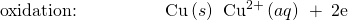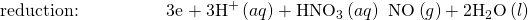6. If necessary, multiply one or both half-reactions so that the number of electrons consumed in one is equal to the number produced in the other.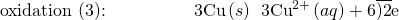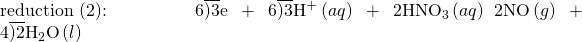7. Add the two half-reactions and simplify.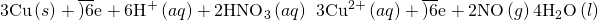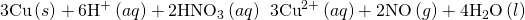8. If the reaction takes place in a basic medium, add OH ions the equation obtained in step 7 to neutralize the H+ ions (add in equal numbers to both sides of the equation) and simplify.
This step not necessary since the solution is stipulated to be acidic.

The balanced equation for the reaction in an acidic solution is then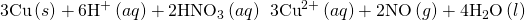Check Your Learning The reaction above results when using relatively diluted nitric acid. If concentrated nitric acid is used, nitrogen dioxide is produced instead of nitrogen monoxide. Write a balanced equation for this reaction.

Answer: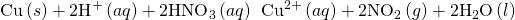Balancing Equations for Redox Reactions in Basic Solutions Write the balanced equation representing reaction between aqueous permanganate ion,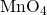, and solid chromium(III) hydroxide, Cr(OH)3, to yield solid manganese(IV) oxide, MnO2, and aqueous chromate ion,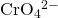The reaction takes place in a basic solution.

Solution Following the steps of the half-reaction method:

1. Write skeletal equations for the oxidation and reduction half-reactions.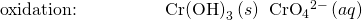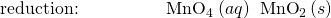2. Balance each half-reaction for all elements except H and O.3. Balance each half-reaction for O by adding H2O.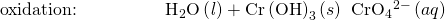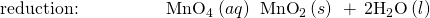4. Balance each half-reaction for H by adding H+.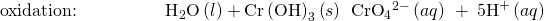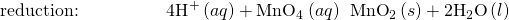5. Balance each half-reaction for charge by adding electrons.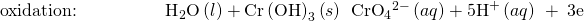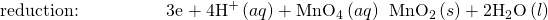6. If necessary, multiply one or both half-reactions so that the number of electrons consumed in one is equal to the number produced in the other.
This step is not necessary since the number of electrons is already in balance.
7. Add the two half-reactions and simplify.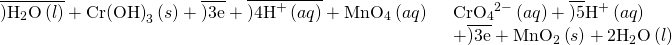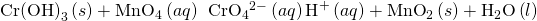8. If the reaction takes place in a basic medium, add OH ions the equation obtained in step 7 to neutralize the H+ ions (add in equal numbers to both sides of the equation) and simplify.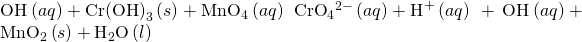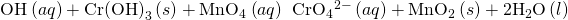Check Your Learning Aqueous permanganate ion may also be reduced using aqueous bromide ion, Br, the products of this reaction being solid manganese(IV) oxide and aqueous bromate ion, BrO3. Write the balanced equation for this reaction occurring in a basic medium.

Answer: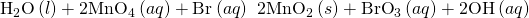### Key Concepts and Summary

Redox reactions are defined by changes in reactant oxidation numbers, and those most relevant to electrochemistry involve actual transfer of electrons. Aqueous phase redox processes often involve water or its characteristic ions, H+ and OH, as reactants in addition to the oxidant and reductant, and equations representing these reactions can be challenging to balance. The half-reaction method is a systematic approach to balancing such equations that involves separate treatment of the oxidation and reduction half-reactions.

### Chemistry End of Chapter Exercises

Identify each half-reaction below as either oxidation or reduction.

(a)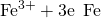(b)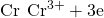(c)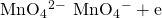(d)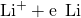(a) reduction; (b) oxidation; (c) oxidation; (d) reduction

Identify each half-reaction below as either oxidation or reduction.

(a)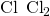(b)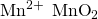(c)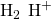(d)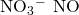Assuming each pair of half-reactions below takes place in an acidic solution, write a balanced equation for the overall reaction.

(a)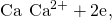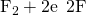(b)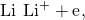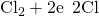(c)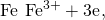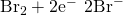(d)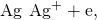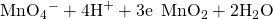(a)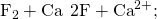(b)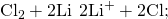(c)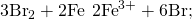(d)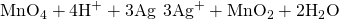Balance the equations below assuming they occur in an acidic solution.

(a)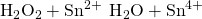(b)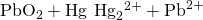(c)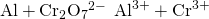Identify the oxidant and reductant of each reaction of the previous exercise.

Oxidized: (a) Sn2+; (b) Hg; (c) Al; reduced: (a) H2O2; (b) PbO2; (c)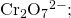oxidizing agent: (a) H2O2; (b) PbO2; (c)reducing agent: (a) Sn2+; (b) Hg; (c) Al

Balance the equations below assuming they occur in a basic solution.

(a)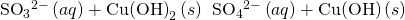(b)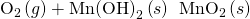(c)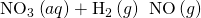(d)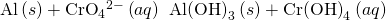Identify the oxidant and reductant of each reaction of the previous exercise.

Oxidized = reducing agent: (a)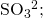(b) Mn(OH)2; (c) H2; (d) Al; reduced = oxidizing agent: (a) Cu(OH)2; (b) O2; (c)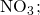(d)Why don’t hydroxide ions appear in equations for half-reactions occurring in acidic solution?

Why don’t hydrogen ions appear in equations for half-reactions occurring in basic solution?

In basic solution, [OH] > 110−7M > [H+]. Hydrogen ion cannot appear as a reactant because its concentration is essentially zero. If it were produced, it would instantly react with the excess hydroxide ion to produce water. Thus, hydrogen ion should not appear as a reactant or product in basic solution.

Why must the charge balance in oxidation-reduction reactions?

### Glossary

electrode potential (EX)
the potential of a cell in which the half-cell of interest acts as a cathode when connected to the standard hydrogen electrode
half cell
component of a cell that contains the redox conjugate pair (“couple”) of a single reactant

## LicenseChemistry 2e by OSCRiceUniversity is licensed under a Creative Commons Attribution 4.0 International License, except where otherwise noted.